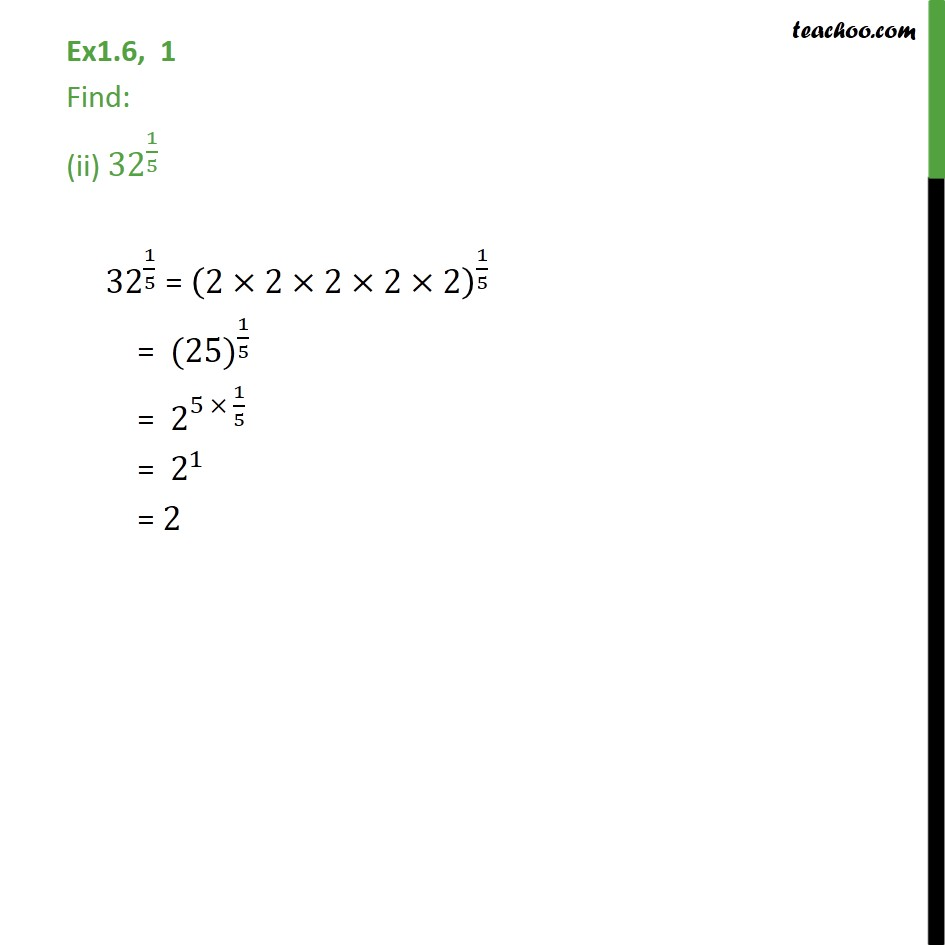1. Chapter 1 Class 9 Number Systems (Term 1)
2. Serial order wise
3. Ex 1.6

Transcript

Ex1.6, 1 Find: (ii) 〖32〗^(1/5) 〖 32〗^(1/5) = (2×2×2×2×2)^(1/5) = 〖 (25)〗^(1/5) = 〖 2〗^(5 × 1/5) = 〖 2〗^1 = 2